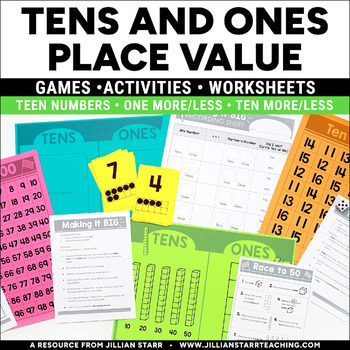Tens and Ones Math CentersK - 2nd
Subjects
Standards
Resource Type
Formats Included
• PDF
Pages
58 pages

Also included in

1. THIS IS A BUNDLED SET OF MY FAVORITE 1ST GRADE MATH ACTIVITIES! Buy them together for 25% less than if you bought them separately!INCLUDED IN THIS BUNDLE:Addition & Subtraction Mega PackOver 100 pages packed with over 20 different math center games and activities! NO FILLER PAGES HERE! This mega
\$55.00
\$71.25
Save \$16.25

Description

Tens and Ones! 55 pages of Common Core aligned games, activities, posters and work pages to teach tens and ones to your students.

Understanding that numbers are composed of tens and ones is a fundamental concept, forming the basis for work with larger numbers. This series of tens and ones games, activities and work pages provides you with 55 pages of resources to teach and support student’s understanding of place value and our base-10 number system.

Tens and Ones Activities Included in this Product:

Race to 50: This game introduces the idea of gathering “ones” and exchanging them for “tens” until you reach 50 (or 5 “tens”). This game uses a mat to illustrate place value, with visual models of tens and ones.

Race to 99/Race to 100: These games teach students the idea of 10 more, 10 less, 1 more and 1 less. Depending on your school’s math program, you can choose either a 100-chart or a 99-chart for your students.

Making It Big: This game strengthens the understanding of place value. Students learn that a 2 in the “ones place” plays a very different role than a 2 in the “tens place”. It also asks students to examine a pair of 2-digit numbers and determine which is greater.

Set of 10-frame playing cards (Numbers 0-9)

Roll, Build & Write: Asks students to roll 2 dice (representing the tens and ones place). Students then build their number with ten-rods and ones. Finally, students are asked to represent their picture with a math sentence.

Ten More: This popular math game asks students to practice mentally adding 10 to a single digit number.

Missing pieces: Asks students to fill in missing numbers from either a 100-Chart or a 99-Chart, depending on your math curriculum.

Set of 24 playing cards: Pairs of cards, one with numbers written numerically, and the other illustrated with ten-rods and ones. (Can be used to play memory, war or Go Fish!)

Tens and Ones Place Value Mat (can be used to reinforce any activity

Ten More/Ten Less: Students roll a dice to determine their starting number (in the teens) and then practice with +10, +20 and -10.

Set of 24 “I have”/”Who has?” Cards: A Tens & Ones version of this well known game helps students practice counting “tens and some more” with visuals.

More & Less: Work Page that asks students to practice adding/subtracting 10 and 1 from given numbers.

Tens & Ones: Work Page that asks students to write the numerical value represented by the ten-rods and ones.

More & Less: 4 Work pages that provides a visual representation of adding and subtracting ten and adding and subtracting one. Students use the visual format of the 0-99 chart or 1-100 to help them identify the “neighbors” of the given numbers. 2 work pages are provided to use with the 0-99 chart, and 2 more are provided to use with the 1-100 chart (depending on your curriculum).

0-99 Chart (for student reference)

1-100 Chart (for student reference)

Number Posters (1-20): Numbers are shown both numerically and with ten frames to illustrate their value.

Meets Common Core Standards:

• CCSS.Math.Content.K.NBT.A.1

• CCSS.Math.Content.1.NBT.A.1

• CCSS.Math.Content.1.NBT.B.2

• CCSS.Math.Content.1.NBT.B.2a

• CCSS.Math.Content.1.NBT.B.2b

• CCSS.Math.Content.1.NBT.B.2c

• CCSS.Math.Content.1.NBT.B.3

• CCSS.Math.Content.1.NBT.C.4

• CCSS.Math.Content.1.NBT.C.5

• CCSS.Math.Content.1.NBT.C.6

OTHER MATH PRODUCTS THAT MAY INTEREST YOU:

Fact Family Bundle

Place Value Bundle-Common Core Aligned Games, Activities & Work Pages

Coin Counting Club- Money Math Center Bundle

Coin Counting Club- Money Math Center Bundle (Canadian Version)

Kindergarten Math Journal Prompts

Complete Set K-4 Prompts

This product is intended for personal use in one classroom only. For use in multiple classrooms, please purchase additional licenses.

Total Pages
58 pages
N/A
Teaching Duration
Lifelong tool
Report this Resource to TpT
Reported resources will be reviewed by our team. Report this resource to let us know if this resource violates TpT’s content guidelines.

Standards

to see state-specific standards (only available in the US).
Subtract multiples of 10 in the range 10-90 from multiples of 10 in the range 10-90 (positive or zero differences), using concrete models or drawings and strategies based on place value, properties of operations, and/or the relationship between addition and subtraction; relate the strategy to a written method and explain the reasoning used.
Given a two-digit number, mentally find 10 more or 10 less than the number, without having to count; explain the reasoning used.
Add within 100, including adding a two-digit number and a one-digit number, and adding a two-digit number and a multiple of 10, using concrete models or drawings and strategies based on place value, properties of operations, and/or the relationship between addition and subtraction; relate the strategy to a written method and explain the reasoning used. Understand that in adding two-digit numbers, one adds tens and tens, ones and ones; and sometimes it is necessary to compose a ten.
Compare two two-digit numbers based on meanings of the tens and ones digits, recording the results of comparisons with the symbols >, =, and <.
The numbers 10, 20, 30, 40, 50, 60, 70, 80, 90 refer to one, two, three, four, five, six, seven, eight, or nine tens (and 0 ones).